Journal of Multimedia Information System
Korea Multimedia Society
Section D

# New chaotic map development and its application in encrypted color image

Abdellatif JarJar1,*
1Moulay Rachid High School, Taza Morocco
*Corresponding Author: Abdellatif JarJar, Moulay Rachid High School, Taza Morocco, abdoujjar@gmail.com

© Copyright 2021 Korea Multimedia Society. This is an Open-Access article distributed under the terms of the Creative Commons Attribution Non-Commercial License (http://creativecommons.org/licenses/by-nc/4.0/) which permits unrestricted non-commercial use, distribution, and reproduction in any medium, provided the original work is properly cited.

Received: Mar 01, 2021; Revised: Jun 09, 2021; Accepted: Jun 15, 2021

Published Online: Jun 30, 2021

## Abstract

This paper traces the process of constructing a new one-dimensional chaotic map, and will provide a simple application in color image encryption. The use of Sarkovskii’s theorem will make it possible to determine the existence of chaos and restrict all conditions to ensure the existence of this new sequence. In addition, the sensitivity to initial conditions will be proved by Lyapunov’s index value. Similarly, the performance of this new chaotic map will be illustrated graphically and compared with other chaotic maps most commonly used in cryptography. Finally, a humble color image encryption application will show the power of this new chaotic map.

Keywords: Chaotic function; Lyapunov’s exponent; Sarkovskii’s theorem

## I. INTRODUCTION

Chaos is a phenomenon very close to randomness, which occupies an important position in cryptography, but determinism and dynamics distinguish it from randomness. In the past three decades, chaos has swept through most sciences (mathematics, physics, biology). It is defined by a nonlinear equation that is very sensitive to initial conditions. The expansion of chaos theory is closely related to the development of computer science and new mathematical advances (modeling, simulation, etc.). Like all new theories, chaos theory is still the subject of many controversies. Various forms of disputes have caused disputes over legal opinions and interpretations. Will science be able to explain it more and more, or is it impossible to understand the world by accident? In fact, for scientists, this is a matter of defining the complexity of the phenomenon they are studying. Chaos as understood by scientists does not mean that there is no order. In fact, this is related to unpredictability, because the final state is very sensitive to the initial state, so long-term evolution cannot be predicted. We believe that the difference between chaos and randomness is the most important point for understanding chaos. Indeed, there is always a tendency to believe that a phenomenon is unpredictable due to the large number of parameters involved. In his description, this prompted us to give a probabilistic method, which by definition can satisfy a certain degree of freedom completely satisfactorily. Randomness. As far as chaos is concerned, this is actually not the case, and the behavior of the chaotic system seems to be random. But in reality, this behavior is described in a deterministic way by fully deterministic nonlinear equations, that is, in particular using mathematics that allow accurate and deterministic methods. To explain with a famous advertisement, a person can write: “Looks like an opportunity, tastes like an opportunity, but not accidental. With the passage of time, people have made several attempts to build a chaotic graph and realized the password. The large number of chaotic graphs used in learning . Other technologies use chaotic maps to construct hash functions . On the other hand, other technologies use chaotic cards in symmetric encryption systems . There are also some the technology combines several chaotic maps to improve performance. Their systems ,, were in the absence of any deterministic formula for generating random numbers, tables of such numbers appeared.

Abreviation

$\begin{array}{c}I=\left[\begin{array}{cc}0& 1\end{array}\right]\\ f:continuous\text{\hspace{0.17em}}function\text{\hspace{0.17em}}over\text{\hspace{0.17em}}I\\ {f}^{k}\left(x\right)=\underset{k\text{\hspace{0.17em}}ins\mathrm{tan}ces}{\underbrace{fofof\dots \text{\hspace{0.17em}}\text{\hspace{0.17em}}\dots \text{\hspace{0.17em}}\text{\hspace{0.17em}}\dots of\left(x\right)}}\\ {G}_{n}=ℤ}{nℤ}Ring\end{array}$
Pseudo-random number generator

Finding that they were unable to master random numbers, researchers quickly turned to the generation of pseudo-random numbers defined by mathematical relationships that produce the same sequence under the same conditions. Among all these technologies, we mentioned the most important ones.

1.1. Von Neumann Generator

In 1946 Von Neumann proposed the following pseudo-random number generator

1. Take an integer (x0) of n digits

2. Calculate $\left({x}_{1}={x}_{0}^{2}\right)$

3. Take the (n) middle digits

4. Restart

1.2. Linear congruence generator

The linear congruential generator was introduced by Lehmer, and is still popular in today’s methods for generating pseudorandom numbers quickly. The sequence of random numbers (xn) is created as follows:

Linear congruence generator

$\begin{array}{c}{x}_{0}\in ℤ}{nℤ}\\ {x}_{n+1}=\left(a{x}_{n}+c\right)\mathrm{mod}\text{\hspace{0.17em}}n\end{array}$
(1)

In order to be able to choose a seed x0 without constraints between 0 andn − 1, it is necessary to try to maximize the generator period. However, it turns out that the values of a and c are known, which makes it possible to obtain a maximum period (equal to n). the period of a linear congruential generator is maximum if and only if:

1. ifc ≠ 0 then c is prime with n.

Namely,it means Pgcd(c,n)=1.

2. For each prime number p dividing n, (a − 1) is a multiple of p.

3. (a − 1)is a multiple of 4 if n is one

1.3. Selected popular chaotic maps

Functions that generate chaotic sequences can be divided into two categories: one-dimensional sequences and multi-dimensional sequences. Chaotic functions are rare in the literature, and there are only a dozen functions used in cryptography.

1.4. One-dimensional chaotic map
1.4.1 Logistic recurrence

Logistic recursion  is a simple example of nonlinear sequence. It is defined by a simple relation managed by a second order polynomial described by the following recurrence relation.

Logistic recurrence

$\begin{array}{l}{\text{u}}_{0}\in \right]0,5\text{\hspace{0.17em}}\text{\hspace{0.17em}}1\left[\text{\hspace{0.17em}}\text{\hspace{0.17em}}\text{\hspace{0.17em}}\text{\hspace{0.17em}}\text{\hspace{0.17em}}\text{\hspace{0.17em}},\text{\hspace{0.17em}}\text{\hspace{0.17em}}\text{μ}\in \left[\begin{array}{cc}3,75& 4\end{array}\right]\hfill \\ {\text{u}}_{\text{n}+1}={\text{μu}}_{\text{n}}\left(1-{\text{u}}_{\text{n}}\right)\hfill \end{array}$
(2)
1.4.2. The Skew Tent Map

The Skew tent map  will be redefined as the equation below

The skew tent map

$\begin{array}{c}{v}_{0}\in \right]0\text{\hspace{0.17em}}\text{\hspace{0.17em}}1\left[\text{\hspace{0.17em}}\text{\hspace{0.17em}}\text{\hspace{0.17em}}\text{\hspace{0.17em}}\text{\hspace{0.17em}}p\in \right]0,5\text{\hspace{0.17em}}\text{\hspace{0.17em}}\text{\hspace{0.17em}}\text{\hspace{0.17em}}1\left[\\ {v}_{n+1}=\left\{\begin{array}{l}\frac{{v}_{n}}{p}\text{\hspace{0.17em}}\text{\hspace{0.17em}}\text{\hspace{0.17em}}\text{\hspace{0.17em}}\text{\hspace{0.17em}}\text{\hspace{0.17em}}\text{\hspace{0.17em}}if\text{\hspace{0.17em}}0\prec {v}_{n}\prec p\hfill \\ \frac{1-{v}_{n}}{1-p}\text{\hspace{0.17em}}\text{\hspace{0.17em}}\text{\hspace{0.17em}}\text{\hspace{0.17em}}if\text{\hspace{0.17em}}p\prec {v}_{n\prec 1}\hfill \end{array}\end{array}$
(3)
1.4.3. PWLCM Map

It is a real linear sequence  by pieces defined by the equation below

PWLCM

$\begin{array}{l}{w}_{n}=f\left({w}_{n-1}\right)\hfill \\ =\left\{\begin{array}{c}\frac{{w}_{n-1}}{d}\text{\hspace{0.17em}}\text{\hspace{0.17em}}\text{\hspace{0.17em}}if\text{\hspace{0.17em}}0\le {w}_{n-d}\le d\\ \begin{array}{l}\frac{{w}_{n-1}-d}{0.5-d}\text{\hspace{0.17em}}\text{\hspace{0.17em}}\text{\hspace{0.17em}}if\text{\hspace{0.17em}}d\le {w}_{n-1}\le 0.5\\ \text{\hspace{0.17em}}\text{\hspace{0.17em}}\text{\hspace{0.17em}}\text{\hspace{0.17em}}\text{\hspace{0.17em}}\text{\hspace{0.17em}}\text{\hspace{0.17em}}\text{\hspace{0.17em}}\text{\hspace{0.17em}}\text{\hspace{0.17em}}\text{\hspace{0.17em}}\text{\hspace{0.17em}}\text{\hspace{0.17em}}f\left(1-{w}_{n-1}\right)\text{\hspace{0.17em}}\text{\hspace{0.17em}}\text{\hspace{0.17em}}\text{\hspace{0.17em}}else\end{array}\end{array}\hfill \end{array}$
(4)

The simplicity and robustness of this card encourages researchers to use it in cryptography.

## II. RECOMMENDED KNOWLEDGE

Before revealing the structure of this new map, it is necessary to define some basic properties. Let (f) be a continuous function over the interval(I) and defined by the equation.

continuous function

$\begin{array}{l}f:I\to I\hfill \\ x\to f\left(x\right)\hfill \end{array}$
(5)

We are going to give some definitions to clarify all the points of the article.

2.1. Trajectory

Let (x) be an element of (I), we call the trajectory of x the set of iterates of (x) by the function (f). This set is defined by

Trajectory

${\Gamma }_{x}=\left\{x,f\left(x\right),{f}^{2}\left(x\right),\text{\hspace{0.17em}}\text{\hspace{0.17em}}\dots \text{\hspace{0.17em}}\text{\hspace{0.17em}}\dots \text{\hspace{0.17em}}\text{\hspace{0.17em}}.\text{\hspace{0.17em}}.\text{\hspace{0.17em}}{f}^{k}\left(x\right),\dots \right\}$
(6)
2.2. Periodicity

We say that xI is periodic if there is an integer (r) such that

Periodicity

$\exists \text{\hspace{0.17em}}r\text{\hspace{0.17em}}\text{\hspace{0.17em}}\in ℕ;{f}^{r}\left(x\right)=x$
(7)

In that case we’ll have

${\Gamma }_{x}=\left\{x,f\left(x\right),{f}^{2}\left(x\right),\text{\hspace{0.17em}}\text{\hspace{0.17em}}\dots \text{\hspace{0.17em}}\text{\hspace{0.17em}}\dots \text{\hspace{0.17em}}\text{\hspace{0.17em}}.\text{\hspace{0.17em}}.\text{\hspace{0.17em}}{f}^{k}\left(x\right),\dots \text{\hspace{0.17em}},{f}^{r-1}\left(x\right)\right\}$
(8)
2.3. Period

The (l) period of an element xI is the smallest integer r such that

Period

(9)

We notice that if (l) is the period of element (x) then

2.4. Transitive topology

Let (f) be a continuous function on I. We say that (f) is topologically transitive if:

Transitive topology

$\forall \left(U,V\right)Ouverts\subset I;\exists \left(x,p\right)\in Uxℕ/{f}^{p}\left(x\right)\in V$
(10)
2.5. Density

It is said that (f) is dense in (I) if:

Density

$\forall \left(x,y\right)\in IxI;\exists \left(\alpha ,p\right)\in Ixℕ/{f}^{p}\left(\alpha \right)\in \left[\begin{array}{cc}x& y\end{array}\right]$
(11)
2.6. Initial Condition Sensitivity

It is said that (f) is sensible to the initial conditions if

Initial condition sensitivity

$\begin{array}{l}\exists \rho >0,\forall \text{\hspace{0.17em}}x\in I,\forall \mu >0,\exists \left(y,p\right)\\ \text{\hspace{0.17em}}\text{\hspace{0.17em}}\text{\hspace{0.17em}}\text{\hspace{0.17em}}\text{\hspace{0.17em}}\text{\hspace{0.17em}}\text{\hspace{0.17em}}\text{\hspace{0.17em}}\text{\hspace{0.17em}}\text{\hspace{0.17em}}\text{\hspace{0.17em}}\text{\hspace{0.17em}}\text{\hspace{0.17em}}\text{\hspace{0.17em}}\text{\hspace{0.17em}}\text{\hspace{0.17em}}\text{\hspace{0.17em}}\text{\hspace{0.17em}}\text{\hspace{0.17em}}\text{\hspace{0.17em}}\text{\hspace{0.17em}}\text{\hspace{0.17em}}\text{\hspace{0.17em}}\in Ixℕ\left\{\begin{array}{c}\begin{array}{l}|x-y|<\mu \\ \text{\hspace{0.17em}}\text{\hspace{0.17em}}\text{\hspace{0.17em}}\text{\hspace{0.17em}}\text{\hspace{0.17em}}\text{\hspace{0.17em}}\text{\hspace{0.17em}}Then\end{array}\\ |{f}^{p}\left(x\right)-{f}^{p}\left(y\right)|>p\end{array}\end{array}$
(12)

In other words,

$For\text{\hspace{0.17em}}\text{\hspace{0.17em}}\rho \text{\hspace{0.17em}}\in \text{\hspace{0.17em}}I\text{\hspace{0.17em}}and\text{\hspace{0.17em}}\text{\hspace{0.17em}}{\rho }^{*}=\rho +\epsilon :\in \sim {10}^{-32}\text{\hspace{0.17em}}then\text{\hspace{0.17em}}{\Gamma }_{\rho \ne }{\Gamma }_{\rho +\in }$
2.7. Fixed points nature
2.7.1. Definition

(x) is a fixed-point if

Fixed point

$\left(f\left(x\right)=x\right)$
(13)

here are two types of fixed points

2.7.2. Attractive fixed point

(x) is an attractive fixed point if and only if

Attractive fixed point

$\exists \left({\alpha }_{n}={f}^{n}\left({\alpha }_{0}\right)\right)that\mathrm{lim}\left({\alpha }_{n}\right)=x$
(14)
2.7.3. repulsive fixed point

(x) is a repulsive fixed point if it is not attractive.

2.7.4. Property

If function (f) is derivable then

Property

$\begin{array}{c}|{f}^{\prime }\left(x\right)|>1\text{\hspace{0.17em}}then\text{\hspace{0.17em}}x\text{\hspace{0.17em}}attractive\text{\hspace{0.17em}}\text{\hspace{0.17em}}fixed\text{\hspace{0.17em}}\text{\hspace{0.17em}}po\mathrm{int}\\ |{f}^{\prime }\left(x\right)|<1\text{\hspace{0.17em}}then\text{\hspace{0.17em}}x\text{\hspace{0.17em}}repulsive\text{\hspace{0.17em}}\text{\hspace{0.17em}}fixed\text{\hspace{0.17em}}\text{\hspace{0.17em}}po\mathrm{int}\\ |{f}^{\prime }\left(x\right)|=1\text{\hspace{0.17em}}then\text{\hspace{0.17em}}ambiguity\end{array}$
(15)

## III. NEW CHAOTIC FUNCTION DESIGN

3.1. Chaotic function
3.1.1. Definition

(f) is a chaotic function if and only if:

the set of periodic points is dense in (I)

1. f is transitive topologically

2. f shows sensitivity to initial conditions

3. Let (f) be a continuous function over the interval I and defined by the equation

Chaotic function design

$\left\{\begin{array}{c}f:\text{\hspace{0.17em}}I\to I\\ Let\text{\hspace{0.17em}}\left(p>0\\ f\left(x\right)=\left\{\begin{array}{c}{p}^{2}x\text{\hspace{0.17em}}\text{\hspace{0.17em}}\text{\hspace{0.17em}}if\text{\hspace{0.17em}}0\le x\le \frac{1}{1+p}\\ p-px\text{\hspace{0.17em}}\text{\hspace{0.17em}}if\text{\hspace{0.17em}}\text{\hspace{0.17em}}\frac{1}{1+p}\le x\le 1\end{array}\end{array}$
(16)
3.2. Graphic representation of the function (f)

The function (f) defined by the equation can be represented by the following figure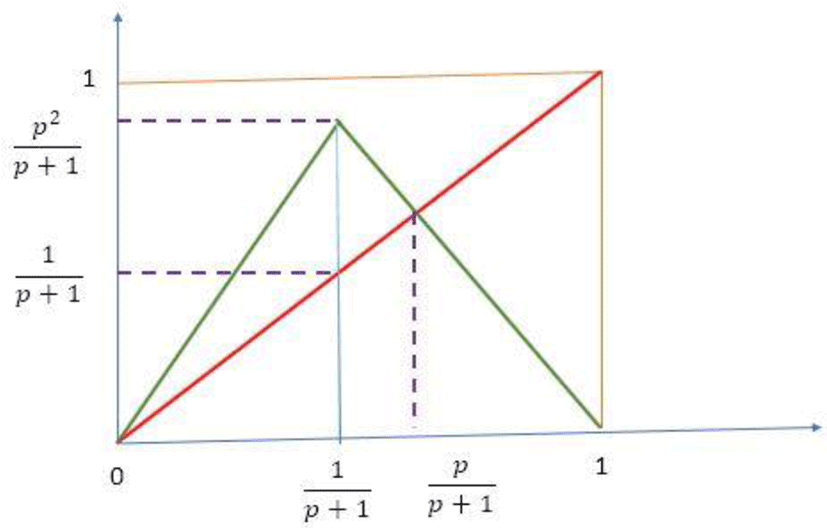Fig. 1. Basic function graph.
3.3. Existence domain of function f

For the sequence (xn) to exist it is necessary that f(I)⊂I. So, it is necessary that

Existence domain

$\left\{\begin{array}{c}\frac{{p}^{2}}{1+p}<1\\ {p}^{2}-p-1<0\end{array}$
(17)
$So\text{\hspace{0.17em}}\text{\hspace{0.17em}}\text{\hspace{0.17em}}\text{\hspace{0.17em}}p\in \left[0\text{\hspace{0.17em}}\text{\hspace{0.17em}}\text{\hspace{0.17em}}\phi \right]$
3.4. Derived from f

The function (f) is continuously derivable and its derivative is given by the expression

Derived from f

$\left\{\begin{array}{l}{f}^{\prime }\left(x\right)={p}^{2}\text{\hspace{0.17em}}\text{\hspace{0.17em}}if\text{\hspace{0.17em}}\text{\hspace{0.17em}}0\le x\le \frac{1}{1+p}\hfill \\ {f}^{\prime }\left(x\right)=-p\text{\hspace{0.17em}}\text{\hspace{0.17em}}if\text{\hspace{0.17em}}\text{\hspace{0.17em}}\frac{1}{1-p}\le x\le 1\hfill \end{array}$
(18)
3.5. (f) fixed points

The two stationary po

(f)fixed point

$\left\{\begin{array}{c}\alpha =0\\ \beta =\frac{p}{1+p}\end{array}$
(19)
3.5.1. Fixed points nature

We have

Fixed point nature

$\left\{\begin{array}{c}|{f}^{\prime }\left(\alpha \right)|={p}^{2}\\ |{f}^{\prime }\left(\beta \right)|=\left\{\begin{array}{l}{p}^{2}\text{\hspace{0.17em}}\text{\hspace{0.17em}}\text{\hspace{0.17em}}\text{\hspace{0.17em}}if\text{\hspace{0.17em}}\text{\hspace{0.17em}}0\le \beta \le \frac{1}{1+p}\hfill \\ p\text{\hspace{0.17em}}\text{\hspace{0.17em}}\text{\hspace{0.17em}}\text{\hspace{0.17em}}if\text{\hspace{0.17em}}\text{\hspace{0.17em}}\frac{1}{1+p}\le \beta \le 1\hfill \end{array}\end{array}$
(20)
$\left\{\begin{array}{l}if\text{\hspace{0.17em}}p<1\text{\hspace{0.17em}}then\text{\hspace{0.17em}}\alpha \text{\hspace{0.17em}}is\text{\hspace{0.17em}}attractive\text{\hspace{0.17em}}fixed\text{\hspace{0.17em}}po\mathrm{int}\hfill \\ if\text{\hspace{0.17em}}p>1\text{\hspace{0.17em}}then\text{\hspace{0.17em}}\alpha \text{\hspace{0.17em}}is\text{\hspace{0.17em}}repulsive\text{\hspace{0.17em}}fixed\text{\hspace{0.17em}}po\mathrm{int}\hfill \end{array}$

Therefore, Preliminary positioning of control parameters (p)

$p\in \left[1\text{\hspace{0.17em}}\text{\hspace{0.17em}}\text{\hspace{0.17em}}\phi \right]$
(21)
3.6. Chaotic sequence building

The sequence (xn) is defined by the following expression

Chaotic sequence building

$\left\{\begin{array}{c}{x}_{0}\in \left[\begin{array}{cc}0& 1\end{array}\right]\text{\hspace{0.17em}}\text{\hspace{0.17em}}\text{\hspace{0.17em}}\text{\hspace{0.17em}}p\in \left[\begin{array}{cc}1& \phi \end{array}\right]\\ f\left({x}_{n}\right)={x}_{n+1}\left\{\begin{array}{l}{p}^{2}{x}_{n}\text{\hspace{0.17em}}\text{\hspace{0.17em}}\text{\hspace{0.17em}}\text{\hspace{0.17em}}if\text{\hspace{0.17em}}\text{\hspace{0.17em}}0\le {x}_{n}\le \frac{1}{1+p}\hfill \\ p-p{x}_{n}\text{\hspace{0.17em}}\text{\hspace{0.17em}}if\text{\hspace{0.17em}}\frac{1}{1+p}\le {x}_{n}\le 1\text{\hspace{0.17em}}\hfill \end{array}\end{array}$
(22)
3.7. Initial Condition Sensitivities

To measure the sensitivity to the initial conditions of the sequence (xn) defined by function (f) , we have to calculate the Lyapunov exponent

Lyapunov exponent

$\lambda =\underset{n\to \infty }{\mathrm{lim}}\left(\frac{1}{n}\right)\sum _{k=0}^{n}Lo{g}_{2}|{f}^{\prime }\left({x}_{k}\right)|$
(23)

In our case, we notice that

$\lambda \gg \underset{n\to \infty }{\mathrm{lim}}\left(\frac{1}{n}\right)\sum _{k=0}^{n}Lo{g}_{2}|{f}^{\prime }\left({x}_{k}\right)|=\underset{n\to \infty }{\mathrm{lim}}\left(\frac{1}{n}\right)\sum _{k=0}^{n}\frac{3}{2}Lo{g}_{2}\left(p\right)\approx \frac{3}{2}Lo{g}_{2}\left(p\right)>0$
(24)

We can conclude from the value of the Lyapunov exponent that the sequence (xn) defined by the function (f) is sensitive to the initial conditions. This value is higher than the value of the logistics diagram, indicating that it is highly sensitive to initial conditions and control parameters.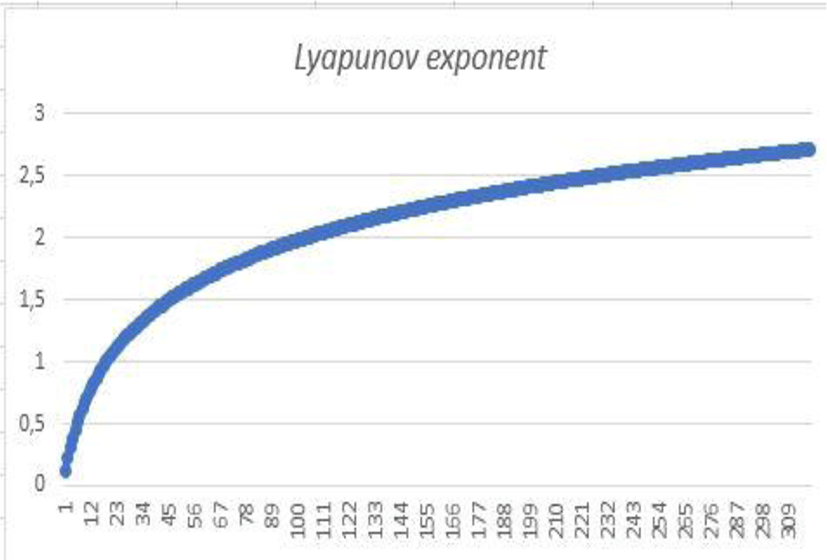Fig. 2. Lyapunov exponent variation with p.

The logarithmic scale plot shows that the distance between two very close initial conditions varies according to an exponential law.

3.8. Sarkovskii’s Theorem

Order of Sarkovkii

Sarkovskii’s Theorem

$\left\{\begin{array}{c}3\succ 5\succ 7\succ 9\succ 11\succ \cdots \text{\hspace{0.17em}}\text{\hspace{0.17em}}\cdots \\ 3\ast 2\succ 5\ast 2\succ 7\ast 2\succ 9\ast 2\succ \cdots \text{\hspace{0.17em}}\text{\hspace{0.17em}}\cdots \\ 3\ast {2}^{2}\succ 5\ast {2}^{2}\succ 7\ast {2}^{2}\succ 9\ast {2}^{2}\succ \cdots \\ ⋮\\ ⋮\\ 3\ast {2}^{2}\succ 5\ast {2}^{2}\succ 7\ast {2}^{n}\succ 9\ast {2}^{n}\succ \cdots \\ ⋮\end{array}$
(25)

All-natural integers are represented in this Sarkovkii order.

The first line represents odd numbers

The row line (n) represents the numbers 2n−1 (2k+1)

3.8.1. Theorem

Let f: II continue. Suppose that (f ) has a periodic point of period ( k). If (k ≻ ℓ) according to Sarkovskii’s order, then f also has a periodic point of period (ℓ).

3.8.2. Corollary (Lie & York)

If (f) admits an item from period 3, then it admits an item of any order. As a result, the function has a chaotic appearance.

Search for

3.8.3. period point 3

Let

Period point 3

$Let\text{\hspace{0.17em}}\text{\hspace{0.17em}}x*=\frac{p}{1+{p}^{5}}\in I$
(26)

Let’s demonstrate that (x⋆) is a point of period 3

Let’s prove that

$\frac{p}{1+{p}^{5}}<\frac{1}{1+p}⇒{p}^{5}-{p}^{2}-p+1>0\text{\hspace{0.17em}}\text{\hspace{0.17em}}\text{\hspace{0.17em}}\text{\hspace{0.17em}}for\text{\hspace{0.17em}}\text{\hspace{0.17em}}\text{\hspace{0.17em}}\text{\hspace{0.17em}}\text{\hspace{0.17em}}p\in \left[\begin{array}{cc}1& \phi \end{array}\right]$
(27)

Let’s put

$f\left(p\right)={p}^{5}-{p}^{2}-p+1$
(28)

Therefore

$\left\{\begin{array}{c}{f}^{\prime }\left(p\right)=5{p}^{4}-2p-1\\ {f}^{″}\left(p\right)=20{p}^{3}-2\text{\hspace{0.17em}}So\text{\hspace{0.17em}}\text{\hspace{0.17em}}{f}^{\left(3\right)}\left(p\right)=60{p}^{3}>0\end{array}$
(29)

The following table gives the variations of the functions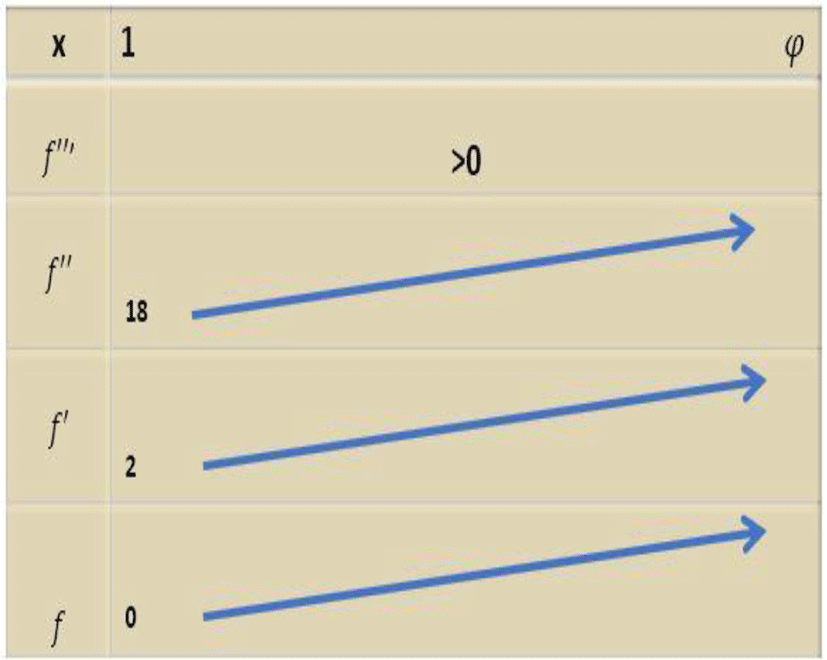Fig. 3. Location of the period 3 point.

So

$\forall p\in \left[\begin{array}{cc}1& \phi \end{array}\right]\frac{p}{1+{p}^{5}}<\frac{1}{1+p}$

Therefore:

$\left\{\begin{array}{c}f\left(x*\right)=\frac{{p}^{3}}{1+{p}^{5}}\\ Let\text{'}s\text{\hspace{0.17em}}\text{\hspace{0.17em}}compare\text{\hspace{0.17em}}\text{\hspace{0.17em}}\frac{{p}^{3}}{1+{p}^{5}}and\frac{1}{1+p}\end{array}$
(30)

Let’s look at the sign of the function (f) defined by

$f\left(p\right)={p}^{5}-{p}^{4}-{p}^{3}+1$
(31)

According to a rough calculation we have

Forp ∈ [1,47 φ] fp) > 0

$\left\{\begin{array}{c}For\text{\hspace{0.17em}}p\in \left[\begin{array}{cc}1,47& \phi \end{array}\right]\text{\hspace{0.17em}}\text{\hspace{0.17em}}\text{\hspace{0.17em}}\text{\hspace{0.17em}}\text{\hspace{0.17em}}We\text{\hspace{0.17em}}\text{\hspace{0.17em}}have\\ f\left(x*\right)=\frac{{p}^{3}}{1+{p}^{5}}\\ {f}^{\left(2\right)}\left(x*\right)=\frac{{p}^{5}}{1+{p}^{5}}>\frac{1}{\left(1+p\right)}\\ {f}^{\left(3\right)}\left(x*\right)=p-p\frac{{p}^{5}}{1+{p}^{5}}=\frac{p}{1+{p}^{5}}=x*\end{array}$
(32)

$x*=\frac{p}{1+{p}^{5}}$ is a periodic point of period 3, therefore (f ) is a chaotic function according to Sarkovskii’s corollary. This period point 3 is illustrated by the following figure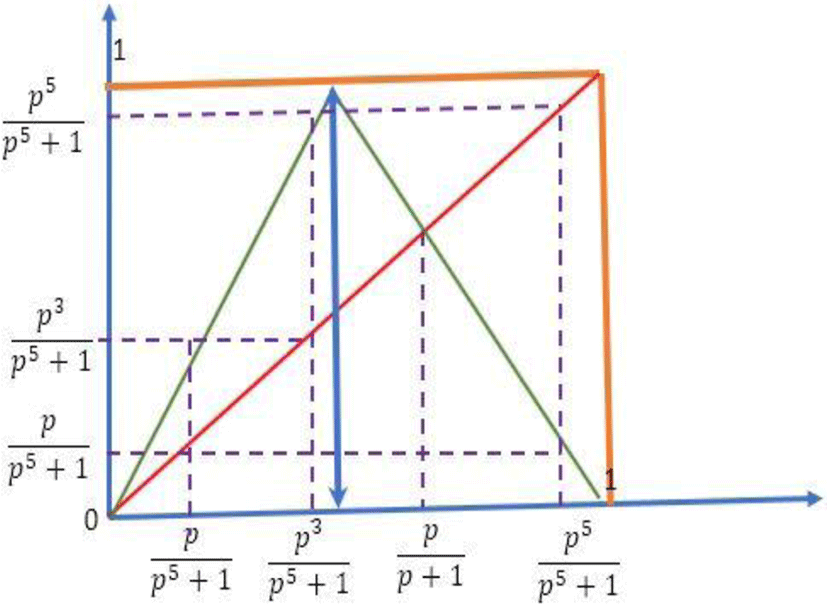Fig. 4. Period point 3.
3.9. Initial sequence values
3.9.1. Period doubling

We know that the only fixed points are

Period doubling

$\left\{\begin{array}{c}\alpha =0\\ \beta =\frac{p}{1+p}\end{array}$
(33)

searches for points (x0) for which there is a k such that

${f}^{k}\left({x}_{0}\right)=\beta$

If there is such a point (xn) then the sequence (xn) is stationary.

For (k=1), we have

$\left\{\begin{array}{c}f\left({x}_{0}\right)=\beta =\frac{p}{1+p}\\ So\\ {x}_{0}=\frac{1}{p\left(1+p\right)}\end{array}$
(34)

For (k = 2), we have

$\left\{\begin{array}{c}{f}^{2}\left({x}_{0}\right)=\beta =\frac{p}{1+p}\\ So\\ {x}_{0}=\frac{1}{{p}^{3}\left(1+p\right)}\end{array}$
(35)

By recurrence, we obtain

For (k = n), we have

$\left\{\begin{array}{c}{f}^{n}\left({x}_{0}\right)=\beta =\frac{p}{1+p}\\ So\\ {x}_{0}=\frac{1}{{p}^{2n-1}\left(1+p\right)}\end{array}$
(36)

If there is (n) such that the initial condition ${x}_{0}=\frac{1}{{p}^{2n-1}\left(1+p\right)}$, then the sequence would be stationary from the n iteration onwards. Then the sequence is no longer chaotic.

We construct a sequence (yn) defined by:

$\left\{\begin{array}{c}n\ge 1\\ We\text{\hspace{0.17em}}have\\ {y}_{n}=\frac{1}{{p}^{2n-1}\left(1+p\right)}\sim \frac{1}{{p}^{2n}}\end{array}$
(37)

(yn) is a decreasing sequence minus 0, converging donations, and we have the following equation;

$For\text{\hspace{0.17em}}\text{\hspace{0.17em}}p>\text{\hspace{0.17em}}\text{\hspace{0.17em}}\underset{n\to \infty }{1\text{\hspace{0.17em}}we\text{\hspace{0.17em}}have\text{\hspace{0.17em}}\mathrm{lim}\left({y}_{n}\right)}=0$
(38)

Finally

$\left\{\begin{array}{c}{x}_{0}>\frac{1}{\left(1+p\right)}\text{\hspace{0.17em}}\text{\hspace{0.17em}}\text{\hspace{0.17em}}\text{\hspace{0.17em}}p\in \left[\begin{array}{cc}1,47& \phi \end{array}\right]\\ f\left({x}_{n}\right)={x}_{n+1}\left\{\begin{array}{l}{p}^{2}{x}_{n}\text{\hspace{0.17em}}\text{\hspace{0.17em}}\text{\hspace{0.17em}}\text{\hspace{0.17em}}if\text{\hspace{0.17em}}\text{\hspace{0.17em}}0\le {x}_{n}\le \frac{1}{1+p}\hfill \\ p-p{x}_{n}\text{\hspace{0.17em}}\text{\hspace{0.17em}}\text{\hspace{0.17em}}if\text{\hspace{0.17em}}\frac{1}{1+p}\le {x}_{n}\le 1\hfill \end{array}\end{array}$
(39)

This is illustrated by the following curve

The sequence (xn) defines is a chaotic sequence under the specified conditions.

We are looking for an element ${x}_{o}>\frac{1}{\left(1+p\right)}$ such as

$\exists \text{\hspace{0.17em}}\text{\hspace{0.17em}}k\text{\hspace{0.17em}}\in ℕ\text{\hspace{0.17em}}\text{\hspace{0.17em}}\text{\hspace{0.17em}}such\text{\hspace{0.17em}}\text{\hspace{0.17em}}as\text{\hspace{0.17em}}f\left({x}_{0}\right)={x}_{k}\frac{1}{{p}^{2k-1}\left(1+p\right)}<\frac{1}{\left(1+p\right)}$
(40)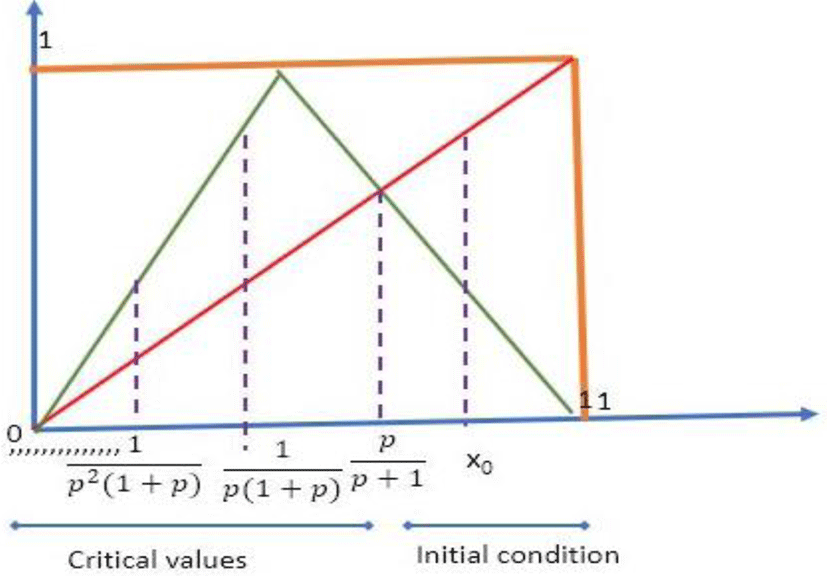Fig. 5. Initial value.

If such a point exists then two situations present themselves

${x}_{0}>\frac{p}{\left(1+p\right)}\text{\hspace{0.17em}}\text{\hspace{0.17em}}\text{\hspace{0.17em}}or\text{\hspace{0.17em}}\text{\hspace{0.17em}}{x}_{0}\in \left[\begin{array}{cc}\frac{1}{\left(1+p\right)}& \frac{p}{\left(1+p\right)}\end{array}\right]$
(41)

Situation 1

$if\text{\hspace{0.17em}}\text{\hspace{0.17em}}{x}_{o}\in \left[\begin{array}{cc}\frac{1}{\left(1+p\right)}& \frac{p}{\left(1+p\right)}\end{array}\right]then\text{\hspace{0.17em}}\text{\hspace{0.17em}}f\left({x}_{0}\right)>\frac{p}{\left(1+p\right)}$
(42)

Situation 2

$if\text{\hspace{0.17em}}\text{\hspace{0.17em}}{x}_{0}>\frac{p}{\left(1+p\right)}\text{\hspace{0.17em}}\text{\hspace{0.17em}}then\text{\hspace{0.17em}}\text{\hspace{0.17em}}\text{\hspace{0.17em}}f\left({x}_{0}\right)<\text{\hspace{0.17em}}\text{\hspace{0.17em}}\frac{p}{\left(1+p\right)}$
(43)

So

$\left\{\begin{array}{c}p-p{x}_{0}=\frac{1}{{p}^{2k-1}\left(1+p\right)}\\ {x}_{0}=\frac{{p}^{2k-1}\left(1+p\right)-1}{{p}^{2k-1}\left(1+p\right)}<\frac{1}{\left(1+p\right)}\end{array}$
(44)

In this case the sequence would be stationary from iteration (k)

${f}^{k}\left({x}_{0}\right)=\frac{p}{1+p}$
(45)

Moreover, we have,

$\forall \text{\hspace{0.17em}}\text{\hspace{0.17em}}k\text{\hspace{0.17em}}\in \text{\hspace{0.17em}}\text{\hspace{0.17em}}ℕ:\frac{{p}^{2k-1}\left(1+p\right)-1}{{p}^{2k-1}\left(1+p\right)}>\frac{p}{1+p}$
(46)

Finally

$\left\{\begin{array}{l}{x}_{0}\in \left[\begin{array}{cc}\frac{1}{\left(1+p\right)}& \frac{p}{\left(1+p\right)}\end{array}\right]\text{\hspace{0.17em}}\text{\hspace{0.17em}}\text{\hspace{0.17em}}\text{\hspace{0.17em}}p\in \left[\begin{array}{cc}1,47& \phi \end{array}\right]\hfill \\ f\left({x}_{n}\right)={x}_{n+1}\left\{\begin{array}{l}{p}^{2}{x}_{n}\text{\hspace{0.17em}}\text{\hspace{0.17em}}\text{\hspace{0.17em}}if\text{\hspace{0.17em}}\text{\hspace{0.17em}}0\le {x}_{n}\le \frac{1}{1+p}\hfill \\ p-p{x}_{n}\text{\hspace{0.17em}}\text{\hspace{0.17em}}\text{\hspace{0.17em}}if\text{\hspace{0.17em}}\text{\hspace{0.17em}}\frac{1}{1+p}\le {x}_{n}\le 1\hfill \end{array}\hfill \end{array}$
(47)

This sequence is chaotic.

3.10. Feigenbaum’s Constants - Renormalization –

In 1975 the physicist Feigenbaum noticed that the general pattern of the logistic sequence was repeated at each bifurcation to within a factor of scale. He then used a process of renormalization. This involves enlarging smaller and smaller parts of the graph and comparing these magnifications to the original pattern. When the enlarged pattern reproduces the first pattern, it is called self-simulation. As it grows to infinity, the general structure repeats itself. If globally, the duplications are not the same, they keep the same ratiosFig. 6. Feigenbaum’s Renormalization.

The first constant intervening horizontally

$\frac{{L}_{1}}{{L}_{2}}\approx \frac{{L}_{2}}{{L}_{3}}\approx 4,57$

The second constant occurring vertically

$\frac{{d}_{1}}{{d}_{2}}\approx \frac{{d}_{2}}{{d}_{3}}\approx 2,5$
3.10.1. Universality

The same sequence, but defined by another function (f) defines single hump type has the same properties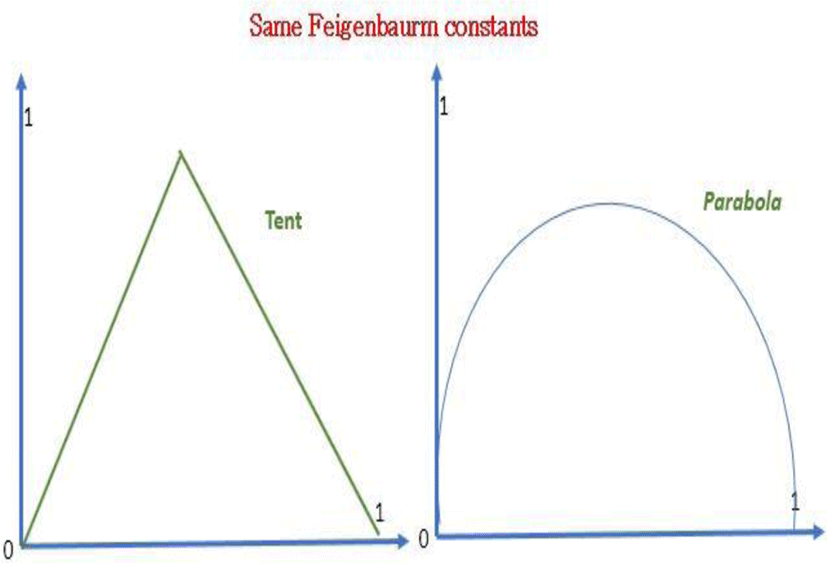Fig. 7. Universality.
3.11. Some simulations
$\left\{\begin{array}{c}{x}_{0}=0,45\\ p=1,5\end{array}$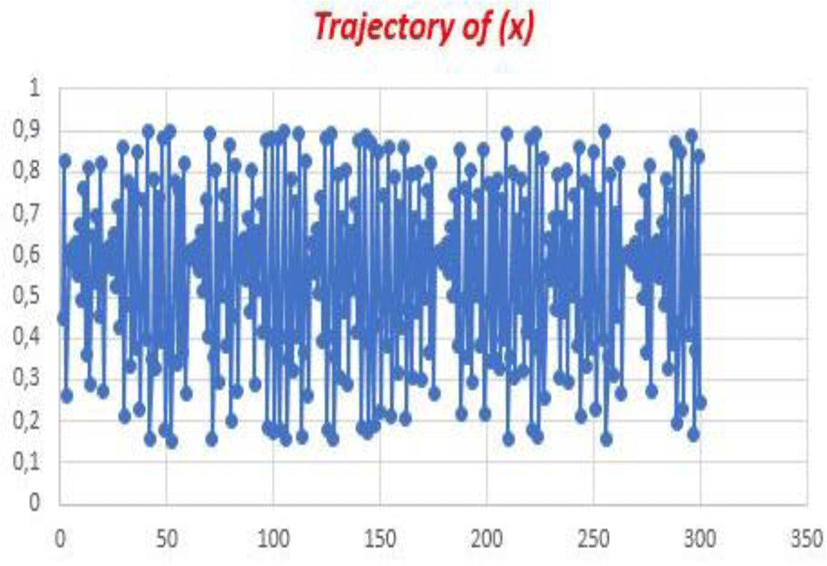Fig. 8. Trajectory of x.

The trajectory seems to be random

3.11.1. Deviation

For two very close values of the initial conditions attached to the same control parameter (p) we see the deviation of the trajectories, this is due to the strong sensitivity to the initial conditions provided by the value of the Lyapunov exponent.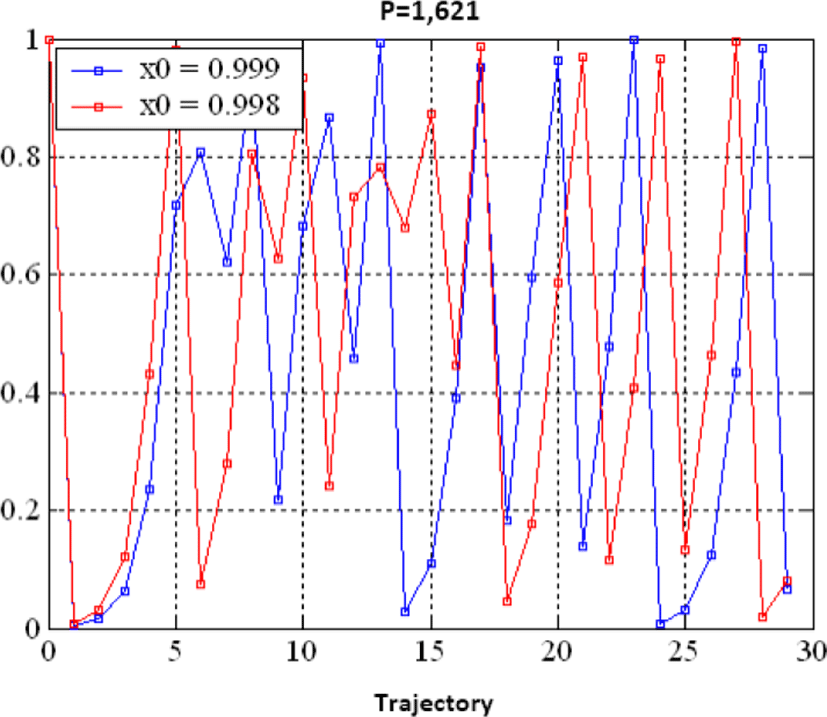Fig. 9. Sensitivity.

We have noticed that small disturbances under initial conditions will greatly deviate from the trajectory.

The sensitivity to initial conditions of a chaotic map measures its robustness against abrupt attacks. Our map is one-dimensional, a comparison with the most used maps is given by the following table.

Table 1. Lyapunov exponent.
Chaotic map Lyapunov exponent
Logistic Map Ln (2)
PWLCM Ln (2)
Tent Map Ln (2)
Our Map 3/2Ln(p)>>Ln(2)

## IV. CRYPTOGRAPHY APPLICATION

We will introduce the improvement of Hill’s classic method using the new chaotic map as the private key to illustrate the performance of our new chaotic map. After reading the original image and switching to the vector, a chaotic vector of the same size is generated from the new map (in the simulation, we take p = 1.54, x0 = 0.623).

4.1. Our algorithm
1. Subdivision of the image vector into blocks of three pixels, as well as the chaotic vector.

2. Calculation of the initialization vector

3. Modification of the priming block

4. Application of Hill’s improved method.

5. Application of dissemination

6. Reconstruction of the encrypted image

7. The encryption process is as follows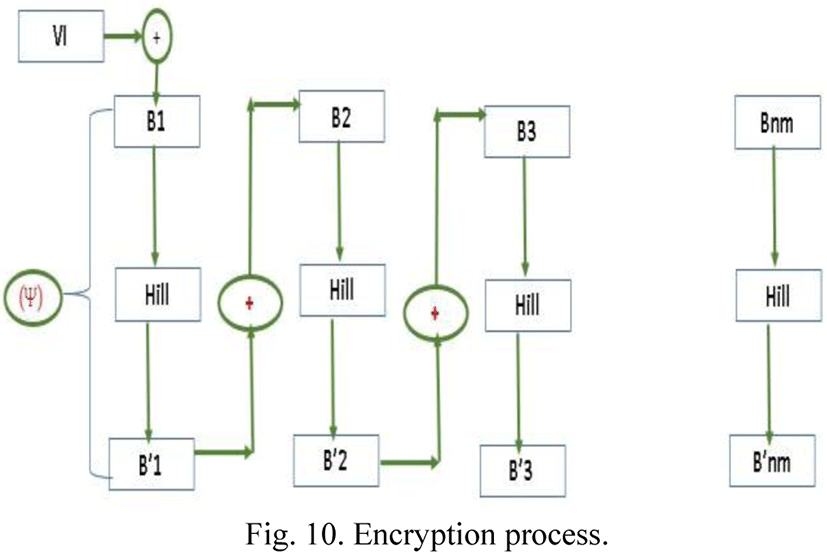Fig. 10. Encryption process.

The encryption function is defined by

Encryption process

$\left\{\begin{array}{c}{B}_{1}=IV\oplus {B}_{1}\\ \Psi \left({B}_{1}\right)={{B}^{\prime }}_{1}=H{B}_{1}\oplus TV1\\ for\text{\hspace{0.17em}}\text{\hspace{0.17em}}i\text{\hspace{0.17em}}\text{\hspace{0.17em}}=2\text{\hspace{0.17em}}to\text{\hspace{0.17em}}nm\\ {B}_{i}={B}_{i-1}\oplus {B}_{i}\\ \Psi \left({B}_{1}\right)={{B}^{\prime }}_{1}=H{B}_{1}\oplus TVi\\ Next\text{\hspace{0.17em}}i\end{array}$
Alg 1

The use of vector (Tvi) aims to overcome the linearity problem of classical systems.

Construct the encryption key matrix from the new chaotic map by the following expression.

$\begin{array}{l}H=\left(\begin{array}{ccc}a& b& c\\ 0& d& e\\ 0& 0& q\end{array}\right)\hfill \\ with\text{\hspace{0.17em}}a,d,q,\epsilon \left(ℤ}{256ℤ}\right)*and\text{\hspace{0.17em}}b,c,eℤ}{256ℤ}\hfill \end{array}$

After the simulation is complete, we get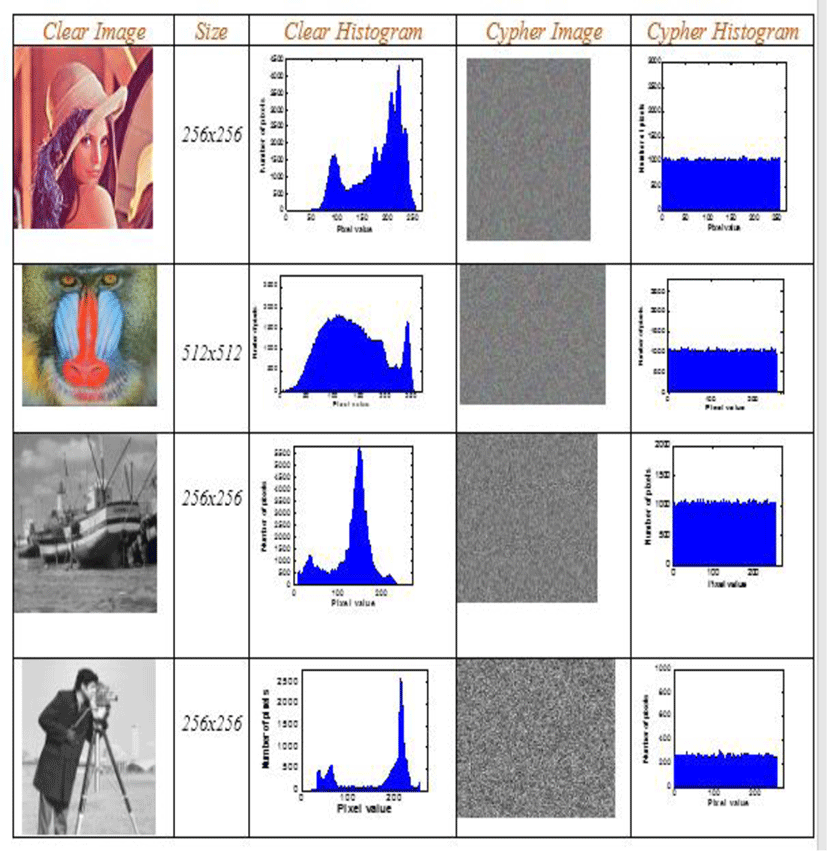Table 2. Encrypted image histogram.

All images tested by our algorithm generate encrypted images with uniform and flattened histograms of pixel distribution. These histograms give an entropy value very close to the maximum value (8). This ensures a strong protection of our new technology against any entropy attack. The calculation of the entropy value is given by the table below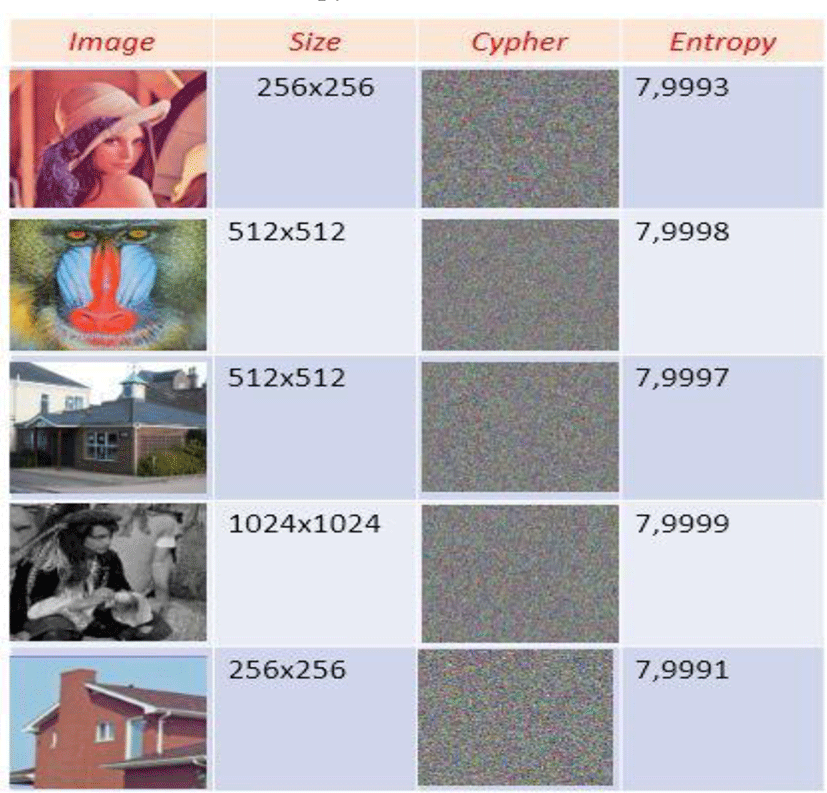Table 3. Calculated entropy.

The use of the encryption mode provides strong protection for our system against differential attacks. The table below illustrates the different values of the differential statistical constants.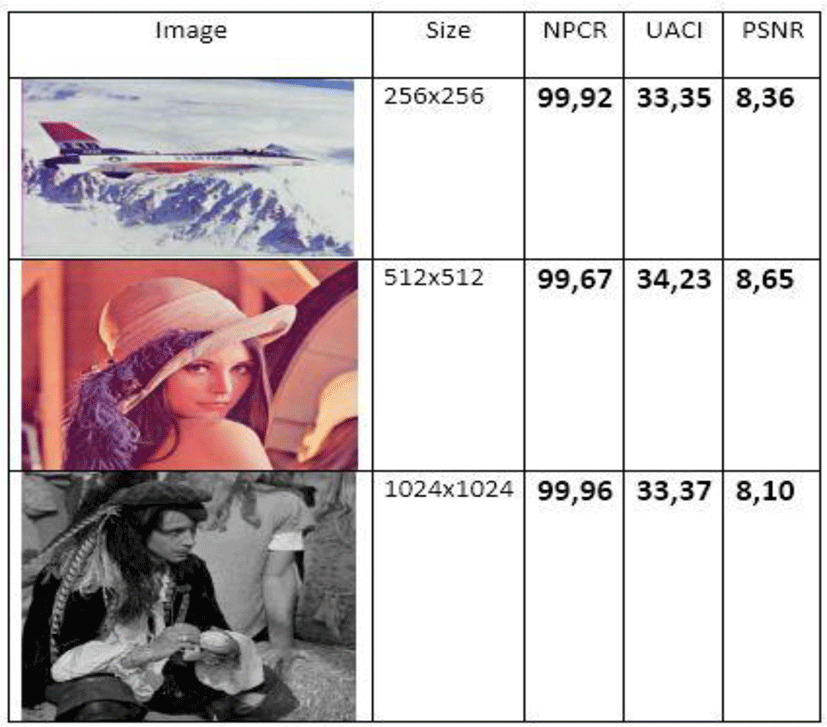Table 4. Differential parameters.
4.1.1. Avalanche effect

Our algorithm uses a strong link between encrypted pixels and pixels with clear policies. As a result, as data propagates through the structure of the algorithm, gradual changes become increasingly important. The avalanche effect is the number of bits that have been changed if a single bit in the original image is changed. The mathematical expression of this avalanche effect is given by

$AE=\left(\frac{\sum {}_{i}bit\text{\hspace{0.17em}}\text{\hspace{0.17em}}change}{\sum {}_{i}bit\text{\hspace{0.17em}}total}\right)*100.$
(48)Table 5. Avalanche effect.

The high sensitivity of our new chaotic map makes our algorithm immune to brute force attacks. This sensitivity is illustrated by the following figure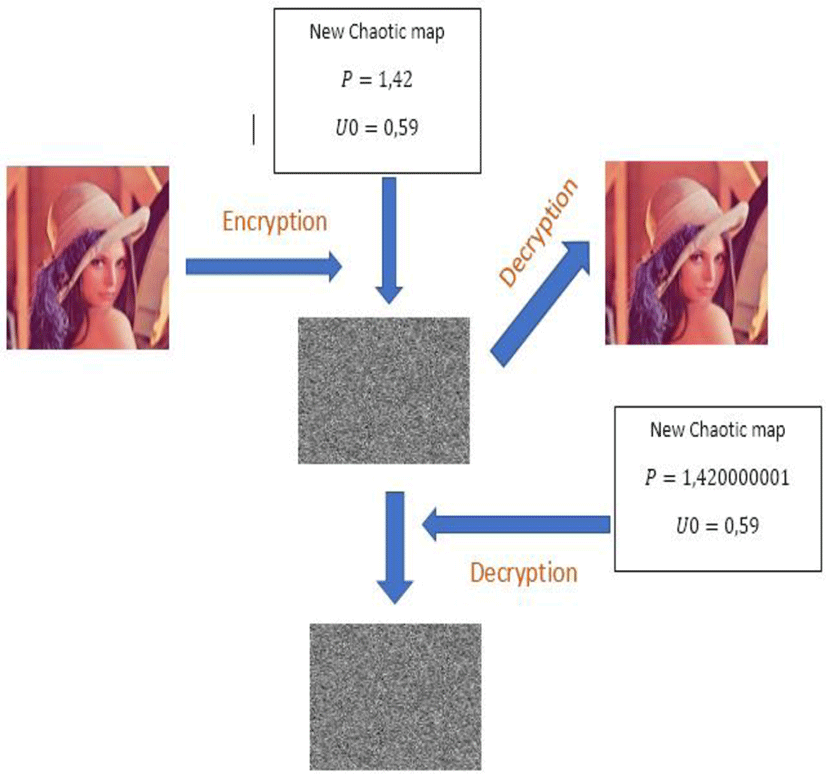Fig. 11. Key sensitivity.

A rapid comparison between our example using a chaotic map with an enhanced Vigenere and other techniques. This minimal comparison, illustrated in the following table, highlights the complexity of our system, devoted by the new chaotic map.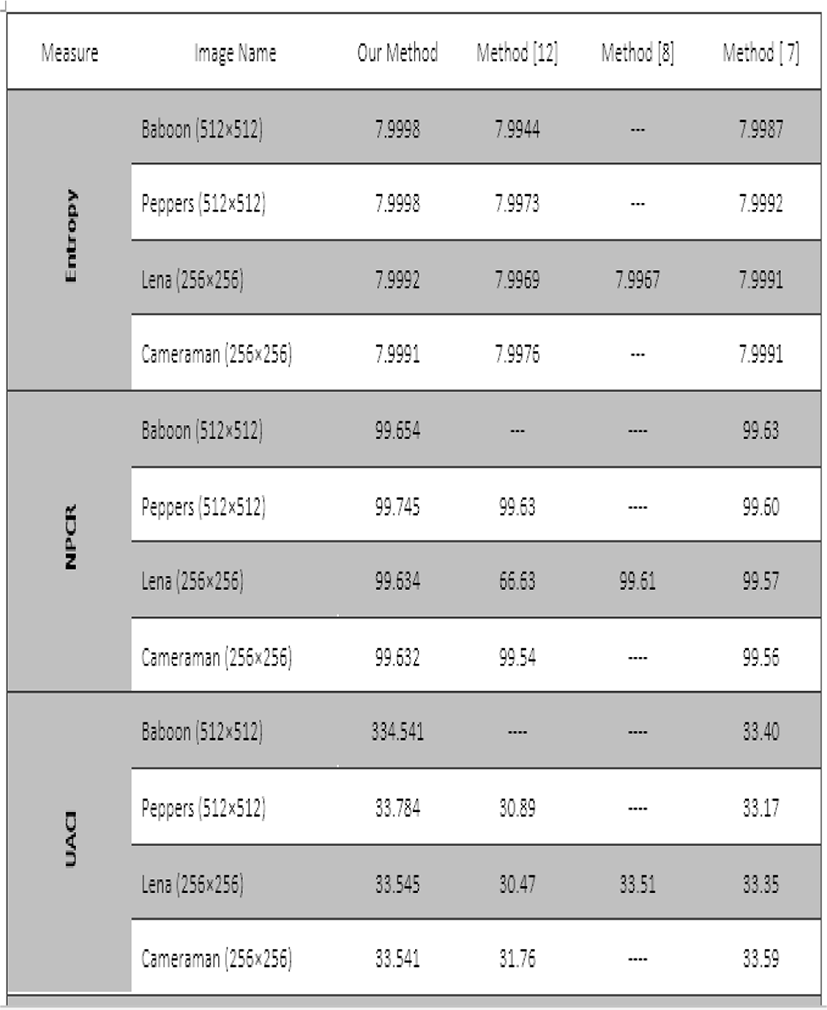Table 6. Comparison of our scheme with other methods.

## V. CONCLUSION

Faced with various difficulties in constructing random numbers, researchers are committed to using generators that follow simple mathematical formulas to create pseudo-random numbers. With the passage of time, chaos theory suddenly appeared, and due to the need to use passwords with such numbers to create private encryption attack. Using logarithms and discrete exponents and translation vectors to overcome linear problems will increase the complexity of our method.

## CONFLICT OF INTEREST

I am the alone author of this article, and therefore no conflict.

To finalize this document, I did not receive any assistance funds from any organization. This document does not contain any studies or experiments on animals.

Ethical approval: This article does not contain any studies with animals performed by any of the authors.

(Or) Ethical approval: This article does not contain any studies with human participants or animals performed by any of the author.

## REFERENCES

.

Günyaz Ablay, “Chaotic Map Construction from Common Nonlinearities and Microcontroller Implementations,” International Journal of Bifurcation and Chaos, vol. 26, no. 7. 2016..

Kwok-Wo Wong, Xiao feng Liao, Yong Wang, Degang Yang, “One-way hash function construction based on chaotic map network,” Chaos, Solitons & Fractals, vol. 41, no. 5, pp. 2566-2574, 2009..

Yantao Li, Shao jiang Deng, and Di Xiao “A novel Hash algorithm construction based on chaotic neural network,” Neural Computing and Applications, vol. 20, pp. 133-141, 2011..

Nicolas Bierne, John, Welch , Etienne Loire , François Bonhomme, and Patrice david, “The coupling hypothesis: why genome scans may fail to map local adaptation genes,” Molecular Ecology, vol. 20, no. 11, pp. 2044–2072, 2011.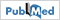.

Jacques Patarin, “Security of Random Feistel Schemes with 5 or More Rounds,” in Proceeding of Annual International Cryptology Conference, pp 106-122, 2004..

Sahar Mazloom, Amir Masud, and Eftekhari-Moghadam, “Color image encryption based on Coupled Nonlinear Chaotic Map,” Chaos, Solitons & Fractals, vol. 42, no. 3, pp. 1745-1754. 2009..

Xiao Feng, Xiaolin Tian, and Shaowe iXia, “An Improved Image Scrambling Algorithm Based On Magic Cube Rotation and Chaotic Sequences,” in Proceeding of IEEE the 4-th International Congress on Image and Signal Processing, pp.1021-1024, 2011.

Hraoui S., Gmira F., Jarar A. O., Satori. K., Saaidi A., “Benchmarking AES and chaos based logistic map for image encryption,” in Proceeding of International Conference on Computer Systems and Applications (AICCSA), pp. 1-4, 2013..

A. Jarjar, “Improvement of hill’s classical method in image cryptography,” International Journal of Statistics and Applied Mathematics, vol. 2, no. 3, Part A, 2017..

A. N. Pisarchik, N. J. Flores-Carmona, and M. Carpio-Valadez, “Encryption and decryption of images with chaotic map lattices,” AIP Chaos, vol. 16, 033118 (2006).

Noshadian S., Ebrahimzade A., and Kazemitabar S. J., “Breaking a chaotic image encryption algorithm,” Multimedia Tools and Applications, vol. 79, no. 35, pp. 25635-25655, 2020..

Niu Y., Zhou Z., and Zhang X., “An image encryption approach based on chaotic maps and genetic operations,” Multimedia Tools and Applications, vol. 79, no. 35, pp. 25613-25633, 2020..

Ghazvini M., Mirzadi M., and Parvar N., “A modified method for image encryption based on chaotic map and genetic algorithm,” Multimedia Tools and Applications, vol. 79, no. 37, pp. 26927-26950, 2020.## Authors

Mr. Abdellatif JarJaris the alone author of this article, and therefore no conflict. To finalize this document, I did not receive any assistance funds from any organization. This document does not contain any studies or experiments on animals. This article does not contain any studies with animals performed by any of the authors. This article does not contain any studies with human participants or animals performed by any of the author.